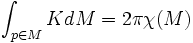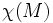# Gauss-Bonnet theorem for surfaces

(diff) ← Older revision | Latest revision (diff) | Newer revision → (diff)
The Gauss-Bonnet theorem states that the average value of Gaussian curvature over a volume-normalized compact orientable two-dimensional Riemannian manifold is proportional to the Euler characteristic of the manifold. Specifically, if$K$ denotes the Gaussian curvature at point$p \in M$,then:$\int_{p \in M} K dM = 2\pi\chi(M)$
Here$\chi(M)$ denotes the Euler characteristic, which is a purely topological notion.